Next: Algorithm Up: Breit-Wheeler Process Previous: Formulas

### Usage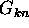are 3-argument functions. In order to avoid discontinuities due to the n-photon threshold, following variables (in addition to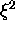) are used as the independent variables instead of (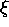,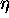, x):

q
Defined by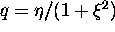. The n-photon threshold is given by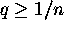.
y
Defined by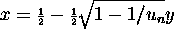.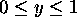represents electrons with energy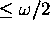and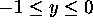those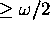. Since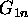and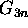are even functions of y, only the part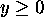is tabulated.

Now, the initialization is done by

C ALL NLBWST(MY,MPH,MXI,MQ,XIMAX,ETAMAX,LENHBW,ENHBWF,IRTN)

MY
Number of y's. (i-th y point is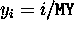,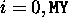)
MPH
Maximum number of laser photons. (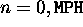)
MXI
Number of's. (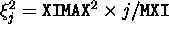,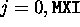)
MQ
Number of q's. Non-equally-spaced MQ points are selected in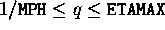.
XIMAX
Maximum.
ETAMAX
Maximum. Must satisfy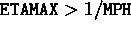. Otherwise, no pair creation is possible.
LENHBW
Flag to apply a rate enhancement function.
ENHBWF
Enhancement function name declared external. Used when LENHCP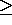1.
IRTN
Return code.
The parameters MY, MPH, MXI can be different from those for nlcpst. A second call of NLBWST will replace the parameters and the arrays created in the first call.

The enhancement function, if needed, has to be defined as

```            FUNCTION ENHBWF(Y)
REAL*8 ENHBWF,Y
ENHBWF=......
RETURN
END```
The enhancement function has to be a function of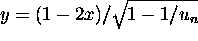(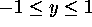) only and it must be an even function of y and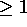for all y. (Actually, only the partis used.) The probability functionsare multiplied by ENHBWF(y). Note that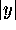close to 1 represents events with a large unbalance of energy between final electron and positron. See NLBWGN for how the weight should be treated in the simulation.

Possible errors 1ex

IRTN=1000
Memory insufficient. You have to reduce MY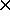MPHMXIMQ or increase the parameter MW in the FORTRAN source.
IRTN=1001 to 1004
Either one of MY,MPH,MXI,MQ is too large.
IRTN=1100
The enhancement function less than 1 at some y.

An event is generated by 1ex

```     NLBWGN(PG,HG,WL,NL,HL,PD,DT,PMAX,IRR,NPH,PELE,HELE,PPOS,HPOS,
PROB,WGT,IRTN)```

Input variables

PG
Array of dimension (0:3). Initial (high energy) photon 4-momentum (eV/c).
HG
Photon helicity. (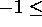HG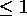).
WL
Laser photon energy (eV).
NL
Array of dimension 3. Unit vector along the laser direction.
HL
Laser helicity (-1 or +1).
PD
Laser power density (Watt/m).
DT
Time interval times the velocity of light (meter).
PMAX
Maximum probability of pair creation in DT. Same as in NLCPGN.
IRR
Random number seed.

Output variables

NPH
If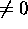, number of absorbed laser photons. If 0, no pair creation.
PELE
Array of dimension (0:3). 4-momentum of final electron.
HELE
Helicity of final electron.
PPOS
Array of dimension (0:3). 4-momentum of final positron.
HPOS
Helicity of final positron.
PROB
Calculated event probability. Same as in NLCPGN.
WGT
Event weight. Same as in NLCPGN.
IRTN
Return code.
The event weight is always 1 if initialized with LENHBW=0. When WGT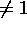(actually,), each of the final pair should be asigned the weight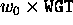and the initial photon still be retained with the weight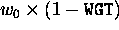, where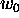is the weight of the initial photon before the event. When WGT=1, the initial photon should be eliminated.

Possible errors

IRTN=1000
Initialization nit yet done.
IRTN=1001is larger than XIMAX.
IRTN=1002is larger than ETAMAX.
IRTN=100
The total rate exceeds PMAX.

Real*8 function

N LBWFN(KK,K,NPH,XI,ETA,X)

returns the value of the function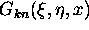, where k=K, n=NPH,=XI,=ETA, and x=X=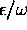. The first argument KK selects either the direct calculation using a Bessel function routine (KK=1) or use interpolation of the stored table (KK=2). In the latter case, initialization must be done in advance and K must be 1 or 3.Next: Algorithm Up: Breit-Wheeler Process Previous: Formulas

Toshiaki Tauchi
Thu Dec 3 17:27:26 JST 1998